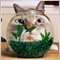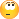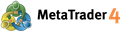How can I get the actual price of each Fibo level ?134

Hi everyone,

When we creat Fibonacci Retracement manually on chart, we can get its level value(50%, 100% etc) as below.

Then how is it possilbe to get the actual price of each level value ?

Thank you X :-)

void OnChartEvent(const int id,
const long& lparam,
const double& dparam,
const string& sparam
)
{
if(id==CHARTEVENT_OBJECT_CREATE)
{
double levelvalue0 = ObjectGetDouble(0,sparam,OBJPROP_LEVELVALUE,0);
double levelvalue1 = ObjectGetDouble(0,sparam,OBJPROP_LEVELVALUE,1);
}
}39055

Good question134

angevoyageur:
Good question

Hi Alain, how have you been! :)

I first tried to get the 0% and 100% price and time but I couldn't get the 0%(=second anchor) one.

datetime time0 = ObjectGetInteger(0,sparam,OBJPROP_TIME,0);
datetime time1 = ObjectGetInteger(0,sparam,OBJPROP_TIME,1);     // -> incorrect value
double  price0 = ObjectGetDouble(0,sparam,OBJPROP_PRICE,0);
double  price1 = ObjectGetDouble(0,sparam,OBJPROP_PRICE,1);     // -> incorrect value
Print("time0=",time0," levelvalue=",levelvalue," price0=",price0," price1=",price1);

I have no idea what to do :(17733

Hi everyone,

When we creat Fibonacci Retracement manually on chart, we can get its level value(50%, 100% etc) as below.

Then how is it possilbe to get the actual price of each level value ?

You can't.  You get the price of the 0% level,  you get the price of the 100% level and when you want the price of the 27.45% level you calculate it using the prices from the 0% and 100% levels.17733

Hi Alain, how have you been! :)

I first tried to get the 0% and 100% price and time but I couldn't get the 0%(=second anchor) one.

I have no idea what to do :(

It works just fine . . .  the attached is a script,  draw a Fib on your chart,  run the script and enter the Fib name.

Files:134

RaptorUK:

It works just fine . . .  the attached is a script,  draw a Fib on your chart,  run the script and enter the Fib name.

Hi Simon,

You are right ! Your script works fine.

Thank you so much.

Have a nice Sunday :)8

RaptorUK:
You can't.  You get the price of the 0% level,  you get the price of the 100% level and when you want the price of the 27.45% level you calculate it using the prices from the 0% and 100% levels.

When you are adding the Fib's manually, indeed it is possible to show the fib-levels AND the inherent price of it.

E.g. if you want to see the price level of the 38.2%-retracement, then add a level in the properties with the parameter 0.382 and

add in the description row 38.2 [%\$]  --> The result will be 38.2 [1.35660] on the fibo line.17733

DonLupo:

When you are adding the Fib's manually, indeed it is possible to show the fib-levels AND the inherent price of it.

E.g. if you want to see the price level of the 38.2%-retracement, then add a level in the properties with the parameter 0.382 and

add in the description row 38.2 [%\$]  --> The result will be 38.2 [1.35660] on the fibo line.

The question was how to get the price of the levels programmatically . . . the answer is you cannot,  they have to be calculated.663

What about getting the actual time on the Time Fibonacci objects -Vertical Fibo- ?5

Don Lupo:

When you are adding the Fib's manually, indeed it is possible to show the fib-levels AND the inherent price of it.

E.g. if you want to see the price level of the 38.2%-retracement, then add a level in the properties with the parameter 0.382 and

add in the description row 38.2 [%\$]  --> The result will be 38.2 [1.35660] on the fibo line.

IT WORKED. thanks for the advice.39055

The object visualization mode for different timeframes can be changed in the "Visualization" tab. The object will then be shown only for the selected timeframes. This can be useful when the tool has different settings for different timeframes. The "Fibo Levels" tab is specifically used only for Fibonacci tools. The list of the tool levels is given here in form of a table. The values of the levels can be changed or deleted (the "Delete" button). A new level can be added by pressing of the "Add" button. At that, if "(%\$)" is entered in the "Description" field, the price value corresponding with this level will be shown in the chart. The "Defaults" button resets the initial values. The "Style" field that allows to set up the color, appearance, and thickness of levels of the object is located in the lower part of the tab.Graphical Objects - Analytics - MetaTrader 4 Help